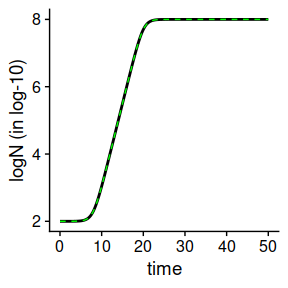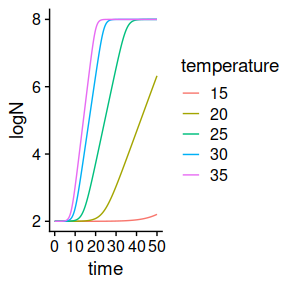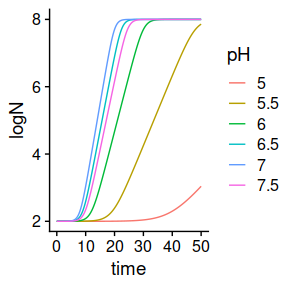# Modelling constant environmental conditions using secondary models

library(biogrowth)
library(tidyverse)
library(cowplot)

The biogrowth package implements two modeling approaches. The first one is based on the use of primary growth models to describe the relationship between the population size and the elapsed time. The second one, expands the primary model considering the effect of changes in the experimental conditions on the specific growth rate (parameter $$\mu$$). This is reflected in the argument environment of predict_growth() (and also fit_growth()), which can take two values: “constant” or “dynamic”. In the first case, the function only takes a primary_model (indeed, passing a secondary_model will return a warning). The logic for this is that the secondary model describes how the environmental conditions affect the kinetic parameters of the primary model (the growth rate). Therefore, the minimum model to describe growth under static conditions is the one that only includes a primary model. Nonetheless, the function can still predict microbial growth under isothermal conditions using environment="dynamic". This can be useful in situations were the environmental conditions are constant, but the response of the population is described using secondary models.

To show this, let’s define an environmental profile where we only consider a constant temperature of 35ºC.

my_conditions <- data.frame(time = c(0, 50),
temperature = c(35, 35)
)

Next, we define primary and secondary models as usual.

q0 <- 1e-4
mu_opt <- .5

my_primary <- list(mu_opt = mu_opt,
Nmax = 1e8,N0 = 1e2,
Q0 = q0)

sec_temperature <- list(model = "CPM",
xmin = 5, xopt = 35, xmax = 40, n = 2)

my_secondary <- list(temperature = sec_temperature)

Finally, we call predict_growth after defining the time points of the simulation.

my_times <- seq(0, 50, length = 1000)

## Do the simulation

dynamic_prediction <- predict_growth(environment = "dynamic",
my_times,
my_primary,
my_secondary,
my_conditions)

Because the temperature during the simulation equals the cardinal parameter $$X_{opt}$$, the predicted population size is identical to the one calculated using predict_growth with environment="constant" for the Baranyi model (calculations for environment="dynamic" are always based on the Baranyi model) when $$\mu = \mu_{opt}$$ and $$\lambda = \frac{ \ln \left(1 +1/Q_0 \right) }{\mu_{opt}}$$.

lambda <- Q0_to_lambda(q0, mu_opt)

primary_model <- list(model = "Baranyi",
logN0 = 2, logNmax = 8, mu = mu_opt, lambda = lambda)

static_prediction <- predict_growth(my_times, primary_model)

plot(static_prediction) +
geom_line(aes(x = time, y = logN), linetype = 2,
data = dynamic_prediction$simulation, colour = "green")The advantages of using a model including a secondary model for modeling growth under constant environmental conditions are evident when simulations are made for several temperatures. Using environment="constant" would require a calculation of the value of $$\mu$$ for each temperature separately. Because the relationship between $$\mu$$ and temperature is included in the secondary model, a separate calculation is not required when using environment="dynamic". max_time <- 100 c(15, 20, 25, 30, 35) %>% # Temperatures for the calculation set_names(., .) %>% map(., # Definition of constant temperature profile ~ data.frame(time = c(0, max_time), temperature = c(., .)) ) %>% map(., # Growth simulation for each temperature ~ predict_growth(environment = "dynamic", my_times, my_primary, my_secondary, env_conditions = ., logbase_mu = 10) ) %>% imap_dfr(., # Extract the simulation ~ mutate(.x$simulation, temperature = .y)
) %>%
ggplot() +
geom_line(aes(x = time, y = logN, colour = temperature)) +
theme_cowplot()Note, however, that predict_growth() does not include any secondary model for the lag phase. The reason for this is that there are no broadly accepted secondary models for the lag phase in predictive microbiology. Therefore, the value of $$\lambda$$ varies among the simulations according to $$\lambda(T) = \frac{ \ln \left(1 +1/Q_0 \right) }{\mu(T)}$$.

Another application of predict_growth() with environment="dynamic" is including the impact of another environmental factor when temperature is kept constant. This can be done by defining a second secondary model.

my_primary <- list(mu_opt = mu_opt,
Nmax = 1e8,N0 = 1e2,
Q0 = q0)

sec_temperature <- list(model = "CPM",
xmin = 5, xopt = 35, xmax = 40, n = 2)

sec_pH <- list(model = "CPM",
xmin = 4, xopt = 7, xmax = 8, n = 2)

my_secondary_2 <- list(temperature = sec_temperature,
pH = sec_pH)

Then, we can call predict_growth().

max_time <- 100

c(5, 5.5, 6, 6.5, 7, 7.5) %>%  # pH values for the calculation
set_names(., .) %>%
map(.,  # Definition of constant temperature profile
~ tibble(time = c(0, max_time),
temperature = c(35, 35),
pH = c(., .))
) %>%
map(.,  # Growth simulation for each temperature
~ predict_growth(environment = "dynamic",
my_times,
my_primary,
my_secondary_2,
env_conditions = .,
logbase_mu = 10)
) %>%
imap_dfr(.,  # Extract the simulation
~  mutate(.x\$simulation, pH = .y)
) %>%
ggplot() +
geom_line(aes(x = time, y = logN, colour = pH)) +
theme_cowplot()As above, note that the lag phase varies between the simulations according to $$\lambda(T, pH) = \frac{ \ln \left(1 +1/Q_0 \right) }{\mu(T, pH)}$$.